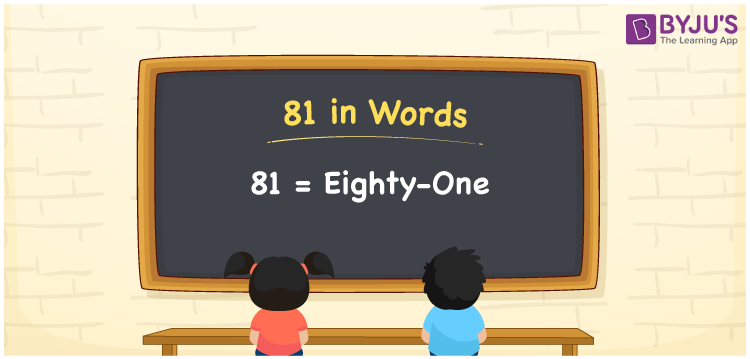# 81 in Words

81 in words is written as Eighty-one. In both the International System of Numerals and the Indian System of Numerals, 81 is written as Eighty-one. The number 81 is a Cardinal Number as it represents some quantity. For example, “there are 81 apples in that basket”, 81 illustrates the number of apples.

 81 in Words Eighty-one Eighty-one in Number 81

## 81 in English Words

We write 81 in English Words using the letters of English alphabets. Therefore, we spell 81 in English as “Eighty-one.”## How to Write 81 in Words?

To write 81 in words, we shall use the place value chart. In the place value chart, write 1 in the ones place and 8 in the tens place. Let us make a place value chart to write the number 81 in words.

 Tens Ones 8 1

Thus, we can write the expanded form as

8 × Ten + 1 × One

= 8 × 10 + 1 × 1

= 80 + 1

= 81

= Eighty-one.

81 is the natural number which is the successor of 80 and predecessor of 82.

81 in words – Eighty-one

• Is 81 an odd number? – Yes
• Is 81 an even number? – No
• Is 81 a perfect square number? – Yes; 9 × 9 = 81
• Is 81 a perfect cube number? – No
• Is 81 a prime number? – No
• Is 81 a composite number? – Yes

## Frequently Asked Questions on 81 in Words

Q1

### How to write 81 in English words?

81 in words is written as Eighty-one.
Q2

### How to spell 81?

The spelling of 81 is “Eighty-one.”
Q3

### Is 81 a Whole Number?

Yes, 81 is a Whole Number.